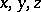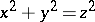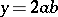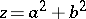# Pythagorean numbers

(diff) ← Older revision | Latest revision (diff) | Newer revision → (diff)
Triplets of positive integerssatisfying. Any solutionto this equation, and consequently all (possibly after switchingonly) Pythagorean numbers, can be expressed as,,, whereandare positive integers. The Pythagorean numbers can be interpreted as the sides of a right-angled triangle (cf. Pythagoras theorem).